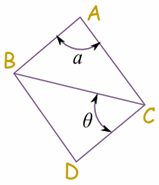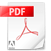Measure the Sides and find the Missing Angle
Find the angle.pdfMeasure the Sides and find the Missing Angle
Find the angle II.pdf

## Names of Angles

### As the Angle Increases, the Name Changes:

 Type of Angle Description Acute Angle an angle that is less than 90° Right Angle an angle that is 90° exactly Obtuse Angle an angle that is greater than 90° but less than 180° Straight Angle an angle that is 180° exactly Reflex Angle an angle that is greater than 180°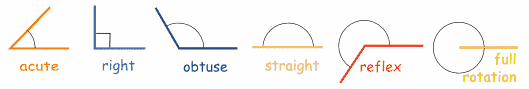### In One Diagram

This diagram might make it easier to remember:

Also: Acute, Obtuse and Reflex are in alphabetical order.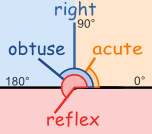## Be Careful What You Measure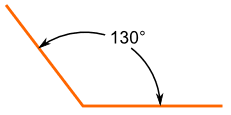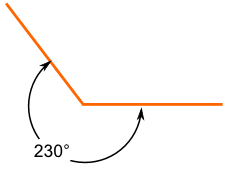This is an Obtuse Angle And this is a Reflex Angle But the lines are the same ... so when naming the angles make sure  that you know which angle is being asked for!

## Positive and Negative Angles

When measuring from a line:

• positive angle goes counterclockwise (opposite direction that clocks go)
• negative angle goes clockwise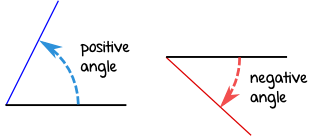### Example: −67°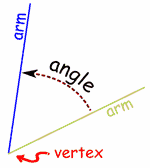## Parts of an Angle

The corner point of an angle is called the vertex

And the two straight sides are called arms

The angle is the amount of turn between each arm.

## How to Label Angles

 There are two main ways to label angles: 1. give the angle a name, usually a lower-case letter like a or b, or sometimes a Greek letter like α (alpha) or θ (theta) 2. or by the three letters on the shape that define the angle, with the middle letter being where the angle actually is (its vertex). Example angle "a" is "BAC", and angle "θ" is "BCD"